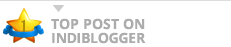# How to calculate Percentage in Excel?Learn how to calculate percentage of nunber against a grant total in Excel & also the reverse. i.e., how do I calculate 10% of a number using plain Excel formula. The post How to calculate Percentage in Excel? appeared first on OfficeTricks.

### Kumarapush

blogs from Coimbatore